# Fusion Plasma Parameters

We are now in a position to estimate the characteristic properties of a thermonuclear plasma trapped in a magnetic confinement device. As we have seen, the critical fusion triple product for achieving nuclear fusion is minimized when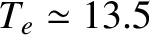keV. Nevertheless, the two burning plasma experiments that are currently under construction, namely the ITER tokamak  and the SPARC tokamak , aim to operate at a somewhat lower volume-averaged plasma temperature of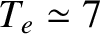keV. Note that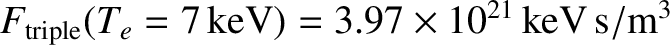. Thus, confinement devices operating at this lower plasma temperature have to satisfy the slightly more stringent Lawson criterion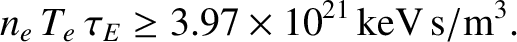(1.21)

The total plasma pressure is written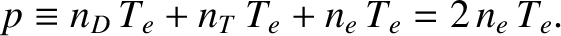(1.22)

Let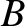be the strength of the magnetic field that confines the plasma. It is helpful to define the dimensionless parameter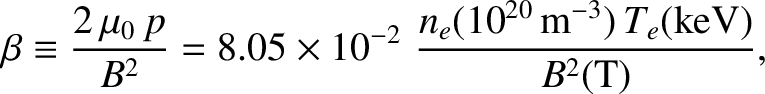(1.23)

which measures the ratio of the plasma thermal energy density to the energy density of the magnetic field. It turns out that plasma stability considerations ensure that conventional magnetic confinement devices, such as tokamaks, cannot safely operate at beta values that exceed a few percent . (See Section 1.10.)

We can write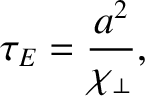(1.24)

where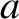is the minor radius of the plasma (i.e., the shortest distance from the plasma core to the plasma boundary), and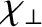is the mean rate at which energy diffuses through the plasma. In practice, energy diffuses out of a magnetic confinement device under the action of small-scale turbulence driven by density and temperature gradients within the plasma [16,64]. Moreover, the typical energy diffusion rate is about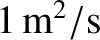.

The previous four equations can be combined to give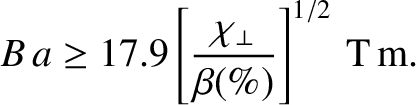(1.25)

Thus, we deduce that, in order to obtain a self-sustaining nuclear fusion reaction in a magnetic confinement device characterized by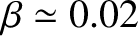[see Equation (1.81)] and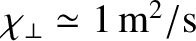, the product of the magnetic field-strength and the plasma minor radius must exceed about 13 tesla-meters. Conventional superconducting magnet technology limits practical magnetic field-strengths in magnetic confinement devices to approximately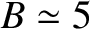T. Thus, to achieve nuclear fusion, such devices must have minor radii of about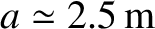. High-temperature superconducting magnet technology allows the practically achievable magnetic field-strength to be increased to approximately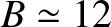T. Thus, to achieve nuclear fusion, high-field devices need only have minor radii of about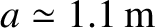, which implies a reduction in the plasma volume by a factor of approximately 12 . This is significant because the cost of a fusion experiment scales roughly as the volume of the experiment.

Table 1.2: Characteristic properties of a thermonuclear plasma trapped in a low-field and a high-field magnetic confinement device. Here,is the magnetic field-strength,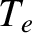the electron temperature,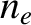the electron number density,the plasma major radius,the plasma minor radius,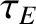the energy confinement time,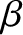the ratio of the thermal energy density to the magnetic energy density, and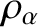the gyro-radius of alpha particle fusion products.
 Low-Field High-Field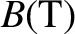5.0 12.0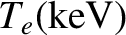7.0 7.0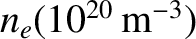0.89 5.1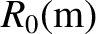7.6 3.2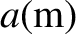2.5 1.1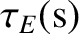6.3 1.10.02 0.02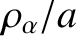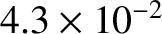The rules of thumb thatand, combined with Equations (1.23)–(1.25), allow us to make the estimates for the characteristic properties of a thermonuclear plasma trapped in both a low-field and a high-field magnetic confinement device that are given in Table 1.2. Note that these estimates accord well with the much more carefully worked out estimates given in References  and . Roughly speaking, thermonuclear fusion requires a deuterium-tritium plasma with a minor radius of at least 1 meter, and an electron number density of at least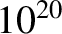particles per cubic meter, to be heated to a mean temperature of about 7 kilo-electronvolts, and the heat to be subsequently confined within the plasma for at least 1 second. The requisite strength of the confining magnetic field is at least 5 telsa.

Of course, in order for magnetic confinement to work properly, the gyro-radii of all of the charged particle species must be significantly less than the minor radius of the plasma. Obviously, the most stringent requirement is that the gyro-radii of alpha particle fusion products,, be less than the minor radius. Now,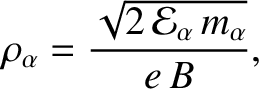(1.26)

where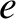is the magnitude of the electron charge . Table 1.2 givesestimates for both low-field and high-field magnetic confinement devices. In both cases,is significantly less than unity, indicating that the magnetic field is, in principle, strong enough to confine all of the charged particles within the plasma. (See, in addition, Table 2.1.)